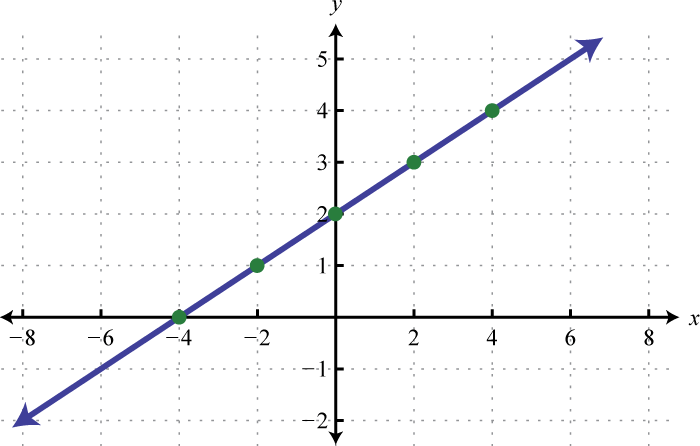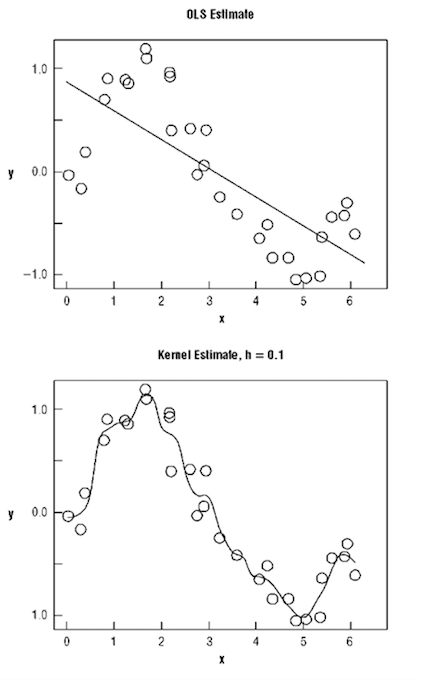# Parametric Model

## What is a Parametric Model?

A Parametric Model is a concept used in statistics to describe a model in which all its information is represented within its parameters. In short, the only information needed to predict future or unknown values from the current value is the parameters. Parametric models often deal with discrete values, whereas non-parametric models will frequently incorporate continuous values and are described as having infinite dimensions.Source

## How does a Parametric Model work?

Parametric models are fairly simple to understand. Imagine a linear regression, in which the straight line of the function is plotted across a graph. Without the graph, one can still predict values using only the parameters, the intercept and the coefficient. With these two parameters, one can predict any value along the regression.Parametric vs. Non-parametric Model
Source

### Parametric vs. Non-Parametric

As mentioned above, parametric models deal with discrete values, and non-parametric models use continuous values. The non-parametric models are also able to predict values of a future state, however in order to do so, the model incorporates information from the current state. Because of their continuous nature, non-parametric models are more flexible and have more degrees of freedom. Put simply, a parametric model can predict future values using only the parameters, but a non-parametric model requires the parameters as well as the current state of the data.